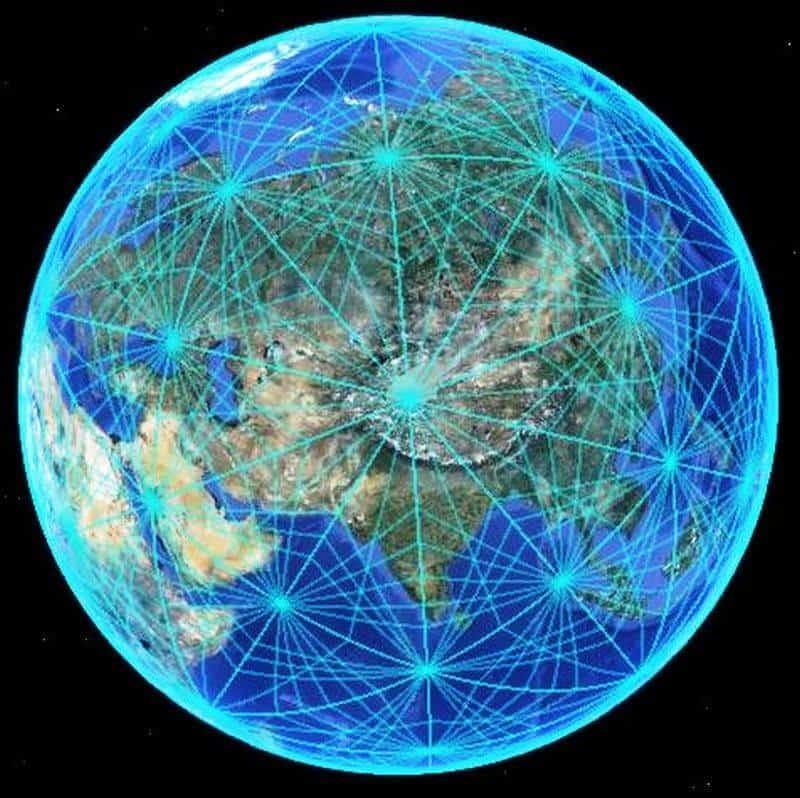# Harmonic 144 is the Speed Of Light

First off, these are not my words or my explanation of the Speed of Light.

Rather, this content comes from Jain 108 and you can find him and his work here: https://www.jain108.com/

I saw this post on Facebook and wanted to make sure that I never lost track of it so that is why I am adding it here to my site.

All credit for this goes to https://www.facebook.com/jainmathemagics/photos/a.235052050013869/356674537851619/?type=3

Photo Credit: ttbtsdisclose.wordpress.com
(Link removed as the site is no longer active)

## Starting With A Circle

Visualize the Circle being divided into 360 degrees.

Similarly, mathematicians like Bruce Cathie, mapped the Earth Grid and observed that the most intelligent way to map 3-dimensional spheres is in Base 60 (our ancient Babylonian heritage, who first invented the Zero or Decimal Place System).

This means, just like the circle of 360 degrees, we can divide each of the 24 Earth hours into 60 minutes, and then divide each minute into seconds, giving 1 Earth Revolution of 24 x 60 x 60 = 86,400 seconds of grid arc.

So using this as our yardstick for measurement, it turns out that the Speed of Light in free space, in geometric terms, has an angular velocity of 144,000 minutes of arc per grid second. In Harmonics, we drop all zeroes and all decimal points and simplify 144,000 to Harmonic 144.

We can also state that the Double Harmonic of the Speed of Light in free space has a value of 144 x 2 = 288 (which I will write about in my next Facebook Post) and that the Half Cycle is 144/2 = 72.

nb: (144×2) = 2c, where c = the speed of light harmonic [E = mc2].

Bruce Cathie called this 24 Hour partitioning of the Full Day as Earth Time and revealed to the world that there exists another Cosmic Time of 27 Hours in a day, which changes all the calculations, but this 27 hr day was the secret knowledge that allowed the precision necessary for dropping nuclear bombs on Hiroshima and Nagasaki (meaning that there is no such thing as Nuclear Attack or Threat, because mathematicians worldwide could, therefore, predict when the next possible bombing could happen according to the trigonometry of the midday sun and the geometric nodal position of the city to be bombed).

In a 27 hour day, there would be 27x60x60 grid seconds per 1 Earth Revolution.
Standard time of 86,400 seconds (24x60x60)
Grid time of 97,200 seconds (27x60x60)
Ratio is 8:9 (86,400 : 97,200)

This ratio of 8:9 is what Squares The Circle approximately.

Of interest, Pythagoras had a whole cosmology based on the Number 27 or 3 cubed.
If this value was applied to the right triangle (3-4-5) of Pythagoras: earth grid ratio (216-288-360)

(3) 216 = 21,600 = number of arc minutes (60 min per degree) in a circle
(5) 360 = 360 = number of degrees in a circle [point activation: earth spins 15°/hour (360/24=15); 30°/bi-hour (360/12=30; zodiac)
(4) 288 = (144×2) = 2c, where c = the speed of light harmonic [E = mc2]

The harmonic of light has a direct relationship with the geometry of the circle; as Pythagoras seems to have been aware.

According to Cathie, the primary grid lines are spaced at intervals of 7.5 minutes of arc N-S/E-W.
If there are 21,600 arc minutes in a circle, which is then divided by 7.5, then a value of 2,880 results; 2,880 harmonically tuned to the speed of light x2 (288)

The only way to traverse the vast distances of space without time is to possess the means of manipulating, or altering, the structure of space; the alteration of the space-time geometric matrix (which provides the illusion of form and distance); alteration of frequencies controlling the matter-antimatter cycles, which govern the perception of space-time structure.

If it was possible to move from one point in space to another (on the grid) in no time (zero-time), then both point positions would coexist in the observer’s reality. Increasing the speed of geometric time decreases space proximity of place (eg. UFO: theoretically travels by means of altering surrounding spatial dimensions for repositioning in space-time; induces zero-point spin via receiving Earth Grid scalar waves transmissions).

All credit for this goes to https://www.facebook.com/jainmathemagics/photos/a.235052050013869/356674537851619/?type=3
Photo Credit: ttbtsdisclose.wordpress.com (Link removed as the site is no longer active)

### 5 thoughts on “Harmonic 144 is the Speed Of Light”

1.Mimi

Mind Blowing. I am trying to figure out why the number 144,000 is important. This goes a long way to explaining it. Thank you!

1.Travis Lee m

Because 144,000 is this. Imagine no zeros exist well first upon what does one billion zeros times 0? Zero right? So.., 1 + 4 = 5 + 4 = 9. Remember the zeros don’t exist!

2.Travis Lee m

3 + 3 is 6 6 * 3 is 18 1 + 8 = 9!!! 9 * 9 is 81 8 + 1 = 9 9 * 3 is 27 2 + 7 = 9.. 3+ 6 = 9.. Jesus was 33 resurrected on third Day